### IMO Shortlist 2011 problem G7

Kvaliteta:
Avg: 0,0
Težina:
Avg: 9,0
Let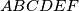$ABCDEF$ be a convex hexagon all of whose sides are tangent to a circle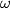$\omega$ with centre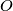$O$. Suppose that the circumcircle of triangle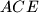$ACE$ is concentric with$\omega$. Let$J$ be the foot of the perpendicular from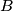$B$ to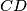$CD$. Suppose that the perpendicular from$B$ to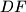$DF$ intersects the line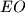$EO$ at a point$K$. Let$L$ be the foot of the perpendicular from$K$ to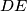$DE$. Prove that$DJ=DL$.

Proposed by Japan
Izvor: Međunarodna matematička olimpijada, shortlist 2011